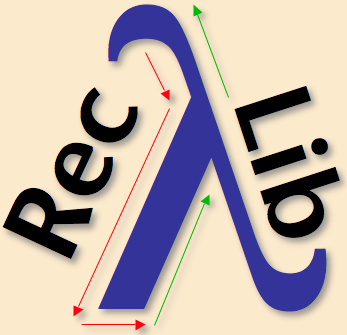RecLib - A Recursion and Traversal Library for Haskell Version: June 2006

The Recursion Library for Haskell provides a rich set of generic traversal strategies to facilitate the flexible specification of generic term traversals. The underlying mechanism is the Scrap Your Boilerplate (SYB) approach. Most of the strategies that are used to implement recursion operators are taken from Stratego.

The library is divided into two layers. The high-level layer defines a universal `traverse` function that can be parameterized by five aspects of a traversal. Also, 96 abbreviations are defined in `Abbr.hs`. Various examples are presented in `Examples.hs`.

The low-level layer provides a set of primitives that can be used for defining more traversal strategies not covered in the library. Two fixpoint strategies inntermost and outermost are defined to demonstrate the usage of the primitives. The design and implementation of the library is explained in the paper listed below. Here are several examples of calling to the higher layer of the library. The examples use the data type of the company example given in the SYB paper.

```increase k =     transformB (always (incS k))
bill       =     accumulate (always col) 0
incBill k  =     acctrans   (always (colS k)) 0
incOne k d = stopTransform  (increase k `mwhenever` isDpt d)
incFst k   = onceTransform  (always (incS k))
incDpt k d =     transformC (mk (\c d -> lookupRate d)) (always incS))
```

## Further Information

A Generic Recursion Toolbox for Haskell, Or: Scrap Your Boilerplate Systematically, Deling Ren and Martin Erwig, ACM SIGPLAN Haskell Workshop, 13-24, 2006